New update is available. Click here to update.

# How To Sort A Stack Using Recursion?

## Introduction

“To understand a sort in recursion, one must first understand recursion.” In data structures and algorithms, we have always come across the popular use of stack i.e., the LIFO data structure. In the real-world application.”

It has proved to be very extensively being used in microprocessors, module semantics handling, data storage in compilation and execution, memory management, etc. In this article, we will be briefly discussing the sorting of elements present in this stack using recursion.

Sorting a stack comes in handy for most of its applications like memory management, storing the context of a process in cases of interrupts and other high priority processes. Sorting can be done iteratively also, although we will see the recursive version here.

## What is Stack?

The stack is a data structure that operates in LIFO fashion i.e. Last in First Out. It contains mainly four basic operations which are being supported top, pushpopsize. The first three operations require constant time while the size operation as expected takes linear time.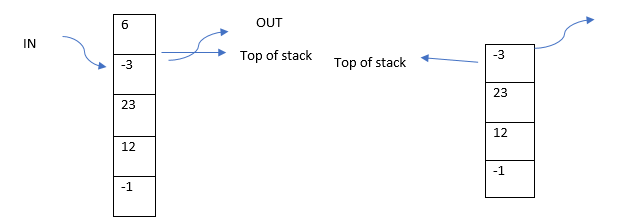Whenever we want to insert into the stack grows upwards, the stack top pointer is updated or incremented and then the new element is inserted. While in popping the stack top pointer is decremented and the element gets out of the stack.

## Operations in Stack

• Push(): Pushes an element into the stack
• Pop(): Pops out the top element of the stack
• Top(): Returns the top element of the stack
• Size(): Returns the size of the stack

## Why Do We Need To Sort A Stack?

As mentioned in the earlier parts stack is a very useful and important data structure that is used in memory management and in the scheduling of process flow. One of the most essential uses of the stack is the program counter stores the context of a processor code to stack whenever it has to switch to a new process so that it can return back to the previous process and complete it after completing the new process.

Now, most of the times these process context needs to be sorted by their priorities for processing and hence sorting has a beneficial application in it.

Now we can do this sorting iteratively too but, in this article, we will be using Recursion.

## Recursion

It’s one of the most important algorithms which makes use of the property that if we can solve a smaller job then we can definitely do the complete job by using the smaller jobs. Recursion basically means to call itself. It has a base case, the main case of handling the smaller problem case and then calling itself for the smaller parts.

It uses the fact that whenever we are standing on a particular state, we assume that our recursive function has completed processing for smaller answers, and now we can combine those to get the answer of our current state.

## Basic Structure of Recursion

``````Void Recursion_function(int somedata){
// Base case
Some condition
//recursive call to itself
Recursion_function( smaller-somedata);
}``````

## How Do We Actually Use Recursion To Sort A Stack?

Well, the idea is quite intuitive and easy. We can recursively pop out each of the elements of the stack and then call a recursive function to insert the elements again in the stack in sorted order.

Understand about Learning Recursion in Python

Let us see the algorithm:

``````While the stack is not empty
Int top = stack top();
Stack pop();
sortStack(); // keep on poping the elements until the stack is empty
sortedInsert( top ); // when the stack is now empty or sorted already inserted in sorted order
SortedInsert() // sorted insert function
If the stack is empty or the stack top < current element
Stack push (current element)
Else {
Top = stack top;
Stack pop();
sortedInsert();
stack push(current element );
}``````

## Visual Working

1. First Iteration keeps on calling the recursive function to pop out the top element until the stack is empty.

• Top element = 6 (Top in the stack frame #1)
• Top element = -3 (Top in the stack frame #2)
• Top element = 23 (Top in the stack frame #3)
• Top element = 12 (Top in the stack frame #4)
• Top element = -1 (Top in the stack frame #5)

2. Now the program gets to the stack frame of the final element i.e. -1 and correspondingly calls the sorted insert function. The stack becomes,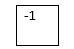3. It gets to the next stack frame i.e. #4 and the current element now is 12, stack top is -1, as 12 > -1, 12 will directly be inserted in the stack.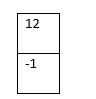4. For the next iteration current element is 23, stack top is 12 hence push it again directly.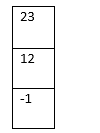5. Now current element is -2 and the stack top is 23 and -2 < 23 hence the new top will be stored and it will recursively call the sortedinsert function with the current -2 until it gets to an element where the stack top is smaller than -2 or the stack becomes empty and then push it.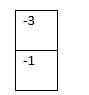6. Push back all the elements in top after the previous element has been placed in its right place.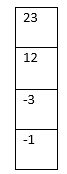7. The above step gets repeated for the next element also and the final stack becomes.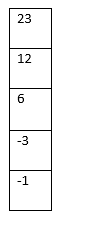We get the final sorted stack.

## Code Implementation

Here I will be using C++ STL to implement a recursive stack.

``````#include<bits/stdc++.h>
using namespace std;
void sortedInsert(stack<int> &st,int element){
if(st.empty() || element > st.top())
st.push(element);
else{
int top_element = st.top();
st.pop();
sortedInsert(st,element);
st.push(top element);
}
}
void sortStack(stack<int> &st){
if(!st.empty()){
int top_element = st.top();
st.pop();
sortStack(st);
sortedInsert(st,top_element);
}
}
int main(){
stack<int> st;
int n;
cin>>n;
while (n--){
int x;
cin>>x;
st.push(x);
}
sortStack(st);
while(!st.empty()){
int top = st.top();
st.pop();
cout<<top<<endl;
}
return 0;
}
Time Complexity: O(n^2)  Space Complexity: O(n) for the auxiliary space stack``````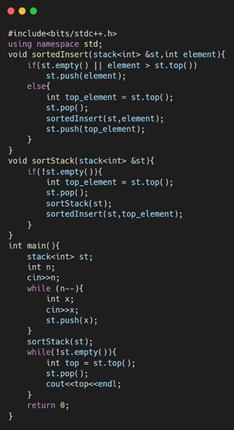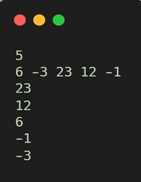### Why do we prefer sorted data?

Organised data is always preferred over random, as it can be more easily analysed and searched for keys more efficiently. It’s always interesting to find out various other sorting techniques and which are the fastest and most preferable ones.

### Why the space complexity of recursion is always at least O(n) ?

Recursion function calls itself multiple times and this is done internally by using function call stack which is again a definitive application of stack. So, this stack again stores some and hence the space complexity is minimum O(n).

### How the time complexity of a recursive function calculated?

Most of the recursive function calls itself multiple times which means for every function call, we will always have the function calls of it’s subproblems. This means for ‘n’ function calls we will have ‘n’ calls again to insert the elements again in this case: O(n^2).

### How do you code recursion?

To see code in recursion, you can look up recursive functions in c, recursive functions in python, recursive functions in java, recursive functions in c++ or recursive functions in data structures in general.

### What is recursion with an example?

Recursion is the phenomenon in programming where a function, called the recursive function calls itself directly or indirectly based on certain conditions.

Example:

void recursion(int n) {

if(n==0) return;

else

recursion(n-1);

}

### What is recursion in language?

Recursion in language is the phenomenon of repeating things in a way that seems similar to the parent thing.

### What is recursion used for?

Recursion is used for breaking down a complex problem into a simpler problem.

### What is recursive thinking?

Recursive thinking is the process of analysing a problem and breaking it down into smaller problems.

### What is recursion syntax?

Recursion syntax is the syntax of writing a recursive call which consists of the name of the recursive call and arguments if any.

## Conclusion

Hope this article has cleared your understanding of stacks and how to sort them using recursion. As we get our results effectively from our code it’s always a good idea to actually code on the machine and practice more such questions, for which do visit the Coding Ninjas’ Code Studio for more such problems.

Recommended Problems:

Hope this will be useful to aspiring programmers and developers.